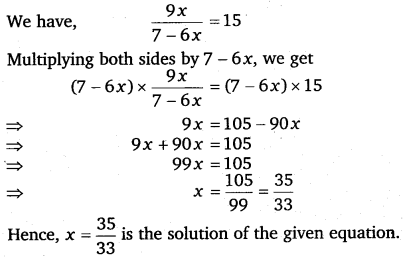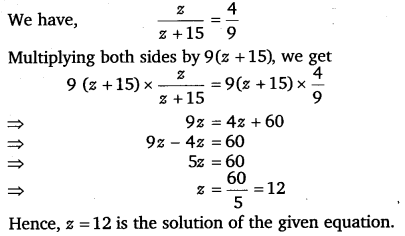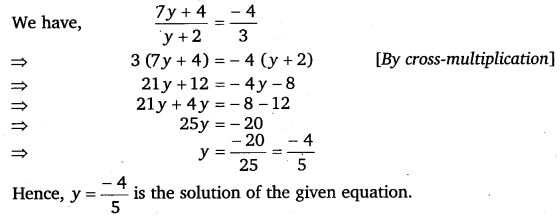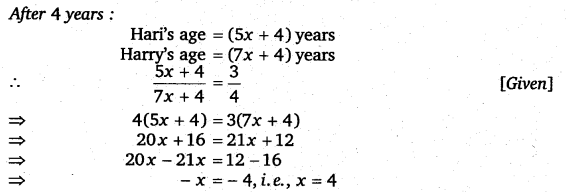# Class 8 Maths NCERT Solutions for Chapter – 2 Linear Equations In One Variable Ex – 2.6

### Linear Equations In One Variable

Question 1.$\frac { 8x-3 }{ 3x } =2$
Solution:Question 2.$\frac { 9x }{ 7-6x } =15$
Solution:Question 3.$\frac { z }{ z+15 } =\frac { 4 }{ 9 }$
Solution:Question 4.$\frac { 3y+4 }{ 2-6y } =\frac { -2 }{ 5 }$
Solution:Question 5.$\frac { 7y+4 }{ y+2 } =\frac { -4 }{ 3 }$
Solution:Question 6.
The ages of Hari and Harry are in the ratio 5: 7. Four years from now the ratio of their ages will be 3 :4. Find their present ages.

Solution.
Let the present ages of Hari and Harry be 5x years and 7x years respectively.∴ Present age of Hari =5 x 4 years = 20 years
∴ Present age of Harry =7 x 4 years = 28 years.

Question 7.
The denominator of a rational number is greater than its numerator by 8. If the numerator is increased by 17 and the denominator is decreased by 1, the number obtained is$\frac { 3 }{ 2 }$. Find the rational number.

Solution.
Let the numerator of the rational number be x. Then, the denominator of the rational number = x + 8.
∴ The rational number =$\frac { x }{ x+8 }$
If the numerator is increased by 17 and the denominator is decreased by 1, the number becomes$\frac { 3 }{ 2 }$.Hence, the required rational number =$\frac { 13 }{ 21 }$.

### Take Your IIT JEE Coaching to Next Level with Examtube

• Mentoring & Teaching by IITians
• Regular Testing & Analysis
• Preparation for Various Engineering Entrance Exams
• Support for School/Board Exams